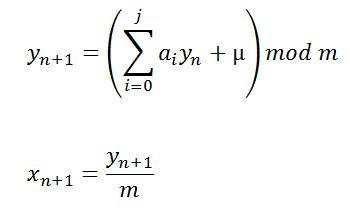# Congruential Generation of Pseudo-Random Numbers in C++

## Congruential Method of Formation of Pseudo-Random Numbers

We need to program a generator of pseudo-random numbers using the congruential method of formation of pseudo-random numbers.

Random numbers means the possible values xi of a continuous random variable X, distributed uniformly on the interval [0;1).

The formula defining the congruential method of generating pseudo-random numbers is represented like this:where, a0, a1, . . . aj — multipliers

µ — increment value

y1, y2, . . . yn — resulting random number

x1, x2, . . . , xn — random numbers on the interval [0;1)

The program generates 15 random numbers, and for the result of the program see below. All the random numbers lie in the interval [0;1).

So considering the formula we should obtain such result:
```0.81 0.13 0.69 0.17 0.01 0.73 0.49 0.57 0.21 0.33 0.29 0.97 0.41 0.93 0.09 0.37 0.61 0.53 0.89 0.77 0.81 0.13 0.69 0.17 0.01 0.73. ```

Let’s try to implement it using C++ tools. All necessary comments will be included:

```#include "stdafx.h"
#include <iostream>
using namespace std;

double pseudo(int &); // prototype for pseudo-random function

int main(int argc, char* argv[])//comand prompt arguments
{
const int amount = 25; // amount of pseudo-random numbers
int x0 = 2; // starting value
cout << "\nPseudo-random:   ";
for (int i = 0; i <= amount; i++)
cout << pseudo(x0) << " "; // generate the i-th number
cout << "\n";
system("pause");
return 0;
}

double pseudo(int &x) // generating pseudo-random numbers function
{
const int a = 100, // generating pseudo-random numbers from 0 to 100
m = 8, // multiplier
increment = 65; // increment value
x = ((m * x) + increment) % a; // out formula
return (x / double(m));
}
```

Let’s try to run the program. Here is what we get:
`Pseudo-random: 0.81 0.13 0.69 0.17 0.01 0.73 0.49 0.57 0.21 0.33 0.29 0.97 0.41 0.93 0.09 0.37 0.61 0.53 0.89 0.77 0.81 0.13 0.69 0.17 0.01 0.73`
Everything works correctly. There are a lot of more complicated ways to implement the solution of this task, but in this guide we concentrated on the most simple one.